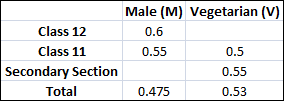### CAT 2007 Question Paper Question 38

Instructions

DIRECTIONS for the following four questions:

The proportion of male students and the proportion of vegetarian students in a school are given below. The school has a total of 800 students, 80% of whom are in the Secondary Section and rest equally divided between Class 11 and 12.Question 38

# What is the percentage of male students in the secondary section?

Solution

According to given condition No of male students in 11,12 and in total are 44 , 48 and 380.

So No of males in secondary are 380-44-48=288.

288 students => 45% of total secondary students.